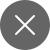# A Brief Guide on Transfer Learning

8+
##### Transfer learning is the most powerful idea in Deep Learning. The name transfer learning itself explains the meaning that it transfers the data (knowledge) it has gained and reuses that knowledge on a completely new problem.

We humans are very good at transferring the knowledge we know from one work to another. Like if we know how to ride a bike then we will use that knowledge while learning car driving. We need to share the knowledge we have and gain by learning. Because no one is born with full knowledge. Transfer learning is quite similar to this.

What exactly is Transfer learning?

Transfer learning is a very popular model in Deep Learning. It stores the knowledge gained after solving a problem and applies that knowledge to a different but similar problem. Transfer learning is a technique that makes machine learning much easier for anyone to apply in real-life. It is a technique where we develop a model for one task and re-use the model as a starting point for an unfamiliar task.

Example: If the Neural network has gained a piece of knowledge by recognizing objects like animals, flowers, fruits, etc. We can use the same knowledge to do better and more useful jobs like reading X-Ray scans, Thermography images, etc. Similarities between the previous tasks and current task processes and content play an important role in the actual world.

Are you thinking about how can we use object recognition data in diagnosis? Don’t worry, I am here to explain to you this. Let’s get more clarity about Transfer Learning.

How Transfer learning works:

Do you remember that early layers of Convolutional neural networks identify simple shapes, edges, etc? And later layers of neural networks recognize more complex patterns, and the very last layer makes predictions. So, most layers from a pre-trained model are useful in new applications because most computer vision problems involve similar low-level patterns like detecting edges, filtering out the noise (Filtering techniques in image processing), etc. We just replace the last layer that makes predictions in our new application.

Let’s say we train a Neural network Now we are training a dataset of images, let it be X and Y is the object we want to categorize our images into. If we want to transfer our knowledge to another application like Radiology diagnosis (X-ray scans), then delete the last neural network layer, which gives output, and insert the additional layer to get the diagnosis predictions.

The knowledge we transfer to the new application can be in various forms depending on the data required and predictions we make out of them.

### Need?

Transfer learning has many benefits when compared to other techniques. The main advantage of transfer learning is it saves training time, no need for large datasets, and it increases the performance of neural networks.

We require a lot of data to train a model and make predictions out of it. Here comes Transfer learning to our rescue. Using Transfer learning we require only less data because we use the model from our previous task.

It has its major uses in Computer vision and Natural language processing. Because in Natural language processing (NLP) we require a tremendous amount of data, if the data is large then processing the data from scratch will take a lot of time which might be in days. To reduce that we will use Transfer learning.

### Definition of Transfer Learning in Mathematics

Now we will see the definition of Transfer Learning in terms of training tasks and domains. Let us assume domain ‘D’ comprising ‘F’ as the feature space and P(X) as the marginal probability distribution. Here specific domain D is D = {F, P(X)} where X = {x1,x2,x3…….} is the subset of F. Every task contains two components, one is label space ‘y’ and the other is function ‘f’ we use for prediction f: F->y. Using function ‘f’ we predict the label (category) f(x) of the instance (image) x. Here instance is the input (x) we consider and using a neural network we try to categorize that as a cat, dog, etc. This categorizing is labeling here.

We denote task as T = {y, f(x)} that will learn from the pre-trained model which comprises pairs {x, y} where y belongs to Y and x belongs to X.

Ds and Dt are the domains corresponding to the source and target tasks. Similarly, Ts and Tt are training tasks of source and target. Where Ds and Dt are different and Ts and Tt are also unequal. Now Transfer learning helps to enhance the training of the target predictive function with the help of the source domain and source training task (Ds and Ts).

How to do Transfer Learning?

If we are training the original data in the tensor flow, then we can restore a few layers for our current task. There are certain algorithms to perform transfer learning in Markov learning and Bayesian networks.

### Applications of Transfer Learning

There are diverse applications of Transfer learning in authentic life. Now let’s see a few of them:

## Conclusion

With Transfer learning, we actually try to know what we have learned in one problem and using that try to improve generalization in another task. With Transfer learning, our life becomes much faster and effortless.